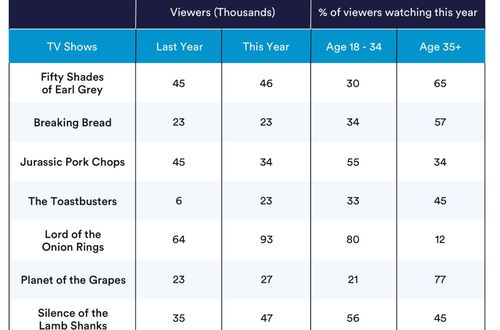# How to answer financial reasoning tests

Applying in a top financial firm or FSA? If yes, then it is important to get familiar with basic tests you need to appear and those are - financial reasoning tests. The tests include 18 financial reasoning and numerical tests, 8 financial and numerical drills, 2 study guides and 11 video tutorials. In this post, we will study what are financial reasoning tests and how to answer in these tests. So, let’s get started…## What are Financial Reasoning Tests?

Financial reasoning tests are equivalent to numerical reasoning tests that present basic financial concepts via graphs, texts and tables. You will receive a short description of a scenario along with extracts of numerical data rather than just receiving a set of tables and charts that contain relevant information to answer the questions.

The mathematical basis of financial reasoning tests is simple arithmetic. It includes 4 basic operations +,-,÷,×, interests, averages, ratios and percentages. The time limit of these tests is usually more than standard numerical tests as they have more textual information that is important to understand the question.

## How to Answer Financial Reasoning Tests?

As mentioned above, financial tests are just like numerical reasoning tests but are more focused on finance. Therefore, the total score depends on the correct answers you give while wrong answers will subtract your points. To answer financial reasoning tests, below are the points you need to consider.

## Step 1: Read the question carefully

While answering the questions, it is important to read the question carefully. Unlike standard numerical tests, data presented in these tests are both numerical and verbal. Each set of questions are accompanied by a passage of 150-200 words.

## Step 2: Be prepared for financial terms and conditions

These tests may have financial concepts and definitions you might not familiar with. Terms like market capitalization, profit margin etc are common in the world of finance, but you can find these words in a more complex way, no matter how simple their mathematical representation is.

## Step 3: More Elaborative calculations

Unlike numerical reasoning tests whose questions need not more than 3-5 mathematical calculations, financial reasoning tests can ask for more elaborate applications and calculations of the latest mathematical expressions.

## Step 4: Psychology is the major factor in these tests

In this test, one of the important roles is played by Psychology. Employers want to see the potential of the candidates to draw conclusion and assumption using logic, even if he or she is not familiar with the specific field.

## Step 5: Take care of the scoring method

The scoring method of this test is not as same as other tests. If you give the wrong answer, your points will be reduced. So while answering the financial reasoning tests, make sure to write a ‘new answer’ choice that allows test takers to say that he doesn’t know the answer.

The scoring method of this test is not as same as other tests. If you give the wrong answer, your points will be reduced. So while answering the financial reasoning tests, make sure to write a ‘new answer’ choice that allows test takers to say that he doesn’t know the answer.

#### Join today

The fastest way to practice

Unlock our complete testing platform and improve faster that ever.